These functions are totally incompatible with the other mechanisms for arranging plots on a device: ‘par(mfrow)’, ‘par(mfcol)’ and ‘split.screen’.

layout()par(mfrow), par(mfcol) 以及 split.screen() 都是完全不相容的。split.screen() 也是一个很灵活的可以分割作图的方法，后面有空再写⚑。

nf <- layout(matrix(c(1, 1, 1,
2, 3, 4,
2, 3, 4), nr = 3, byrow = TRUE))
hist(rnorm(25), col = "VioletRed")
hist(rnorm(25), col = "VioletRed")
hist(rnorm(25), col = "VioletRed")
hist(rnorm(25), col = "VioletRed")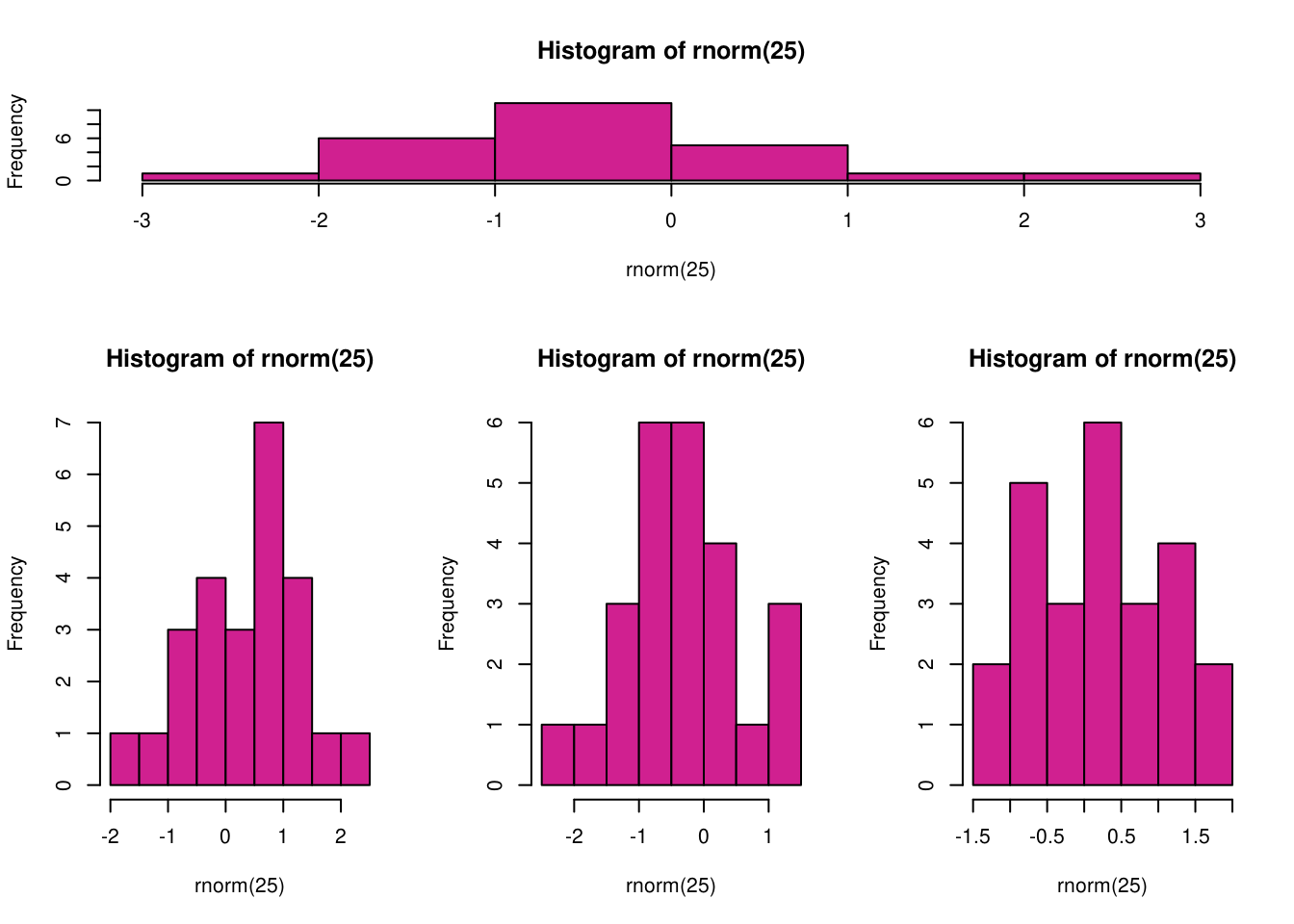layout() 语法不了解的话初看起来完全看不懂，但是其实学习了之后就会发现既简单又灵活。它最主要的是接受一个 matrix 来定义图形布局。比如在上面的例子里我们定义了一个 3 x 3 的矩阵，矩阵数字只有 1/2/3/4。这个数字就是编号，表示整幅图会有 4 个小图。现在再把这个 3 x 3 矩阵想象成一个图片，整个图片被分成 3 行 3 列一共 9 个小格子，然后要画的 4 个小图怎么排布到 9 个小格子呢？看矩阵！矩阵里相应位置是几就放第几幅小图。有的位置有重复的话就表示一幅小图占用了不止一个小格子的位置。所以回过头再看上面的例子，我们定义的 3 行 3 列的布局里，第一行都是 1，表示第一幅图会占据这个图片第一行的位置，依此类推，下面的第二、三行都是一个小图占用两行的高度和 1/3 列的宽度。这样这个图片的布局就一目了然了。

layout() 也支持查看布局：

nf <- layout(matrix(c(1, 1, 1,
2, 3, 4,
2, 3, 4), nr = 3, byrow = TRUE))
layout.show(nf)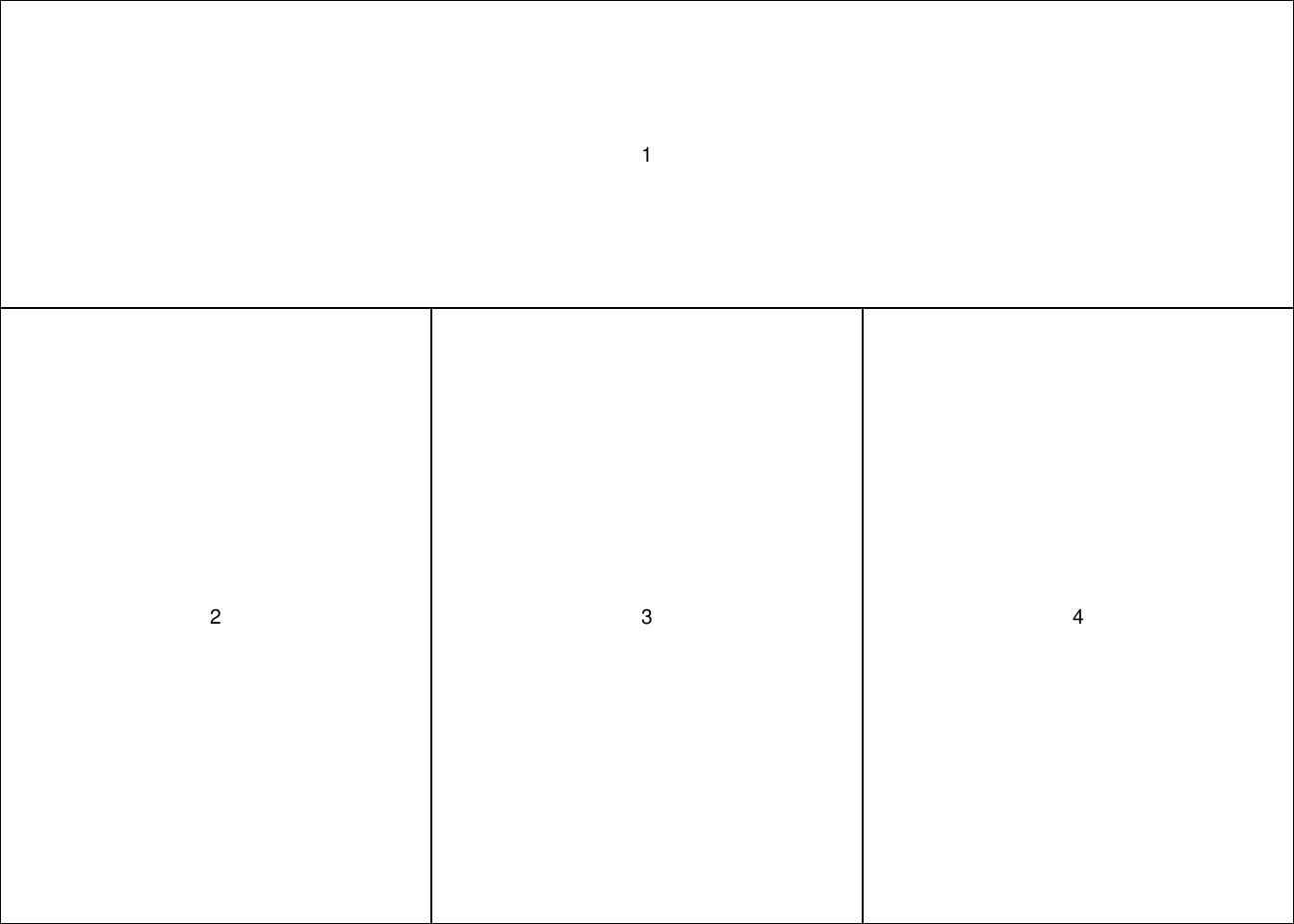nf <- layout(matrix(c(1, 1, 1,
2, 3, 4), nr = 2, byrow = TRUE),
widths = c(1, 1, 1), heights = c(1, 2))
layout.show(nf)set.seed(100)
n <- 100
x <- pmin(3, pmax(-3, stats::rnorm(n)))
y <- pmin(3, pmax(-3, stats::rnorm(n)))
xhist <- hist(x, breaks = seq(-3, 3, 0.5), plot = FALSE)
yhist <- hist(y, breaks = seq(-3, 3, 0.5), plot = FALSE)
top <- max(c(xhist$counts, yhist$counts))
xrange <- c(-3, 3)
yrange <- c(-3, 3)
nf <- layout(matrix(c(2, 0, 1, 3),
2, 2, byrow = TRUE),
c(3, 1), c(1, 3), TRUE)
layout.show(nf)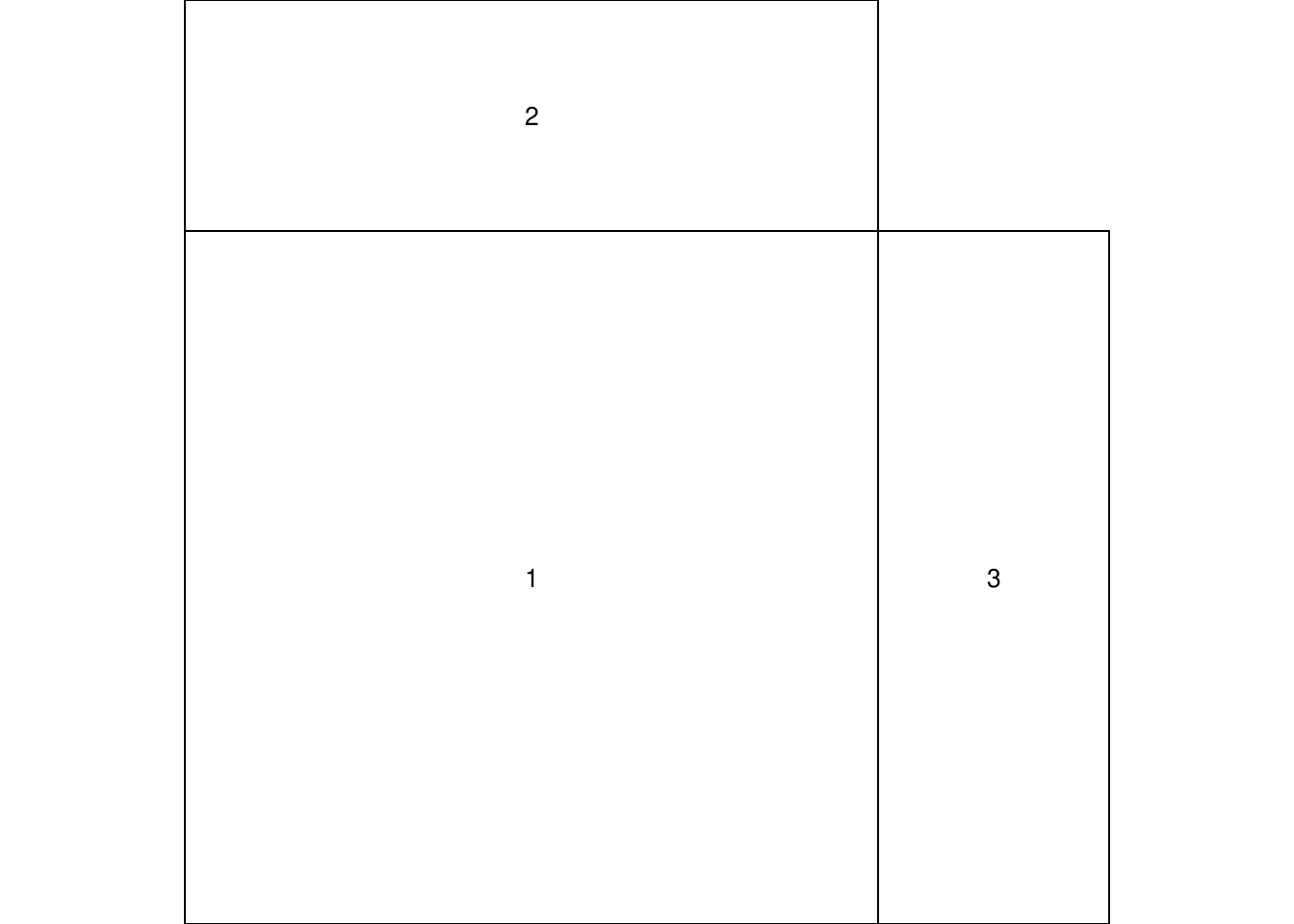par(mar = c(3, 3, 1, 1))
plot(x, y,
xlim = xrange, ylim = yrange,
xlab = "", ylab = "")
par(mar = c(0, 3, 1, 1))
barplot(xhist$counts, axes = FALSE, ylim = c(0, top), space = 0) par(mar = c(3, 0, 1, 1)) barplot(yhist$counts, axes = FALSE,
xlim = c(0, top), space = 0,
horiz = TRUE)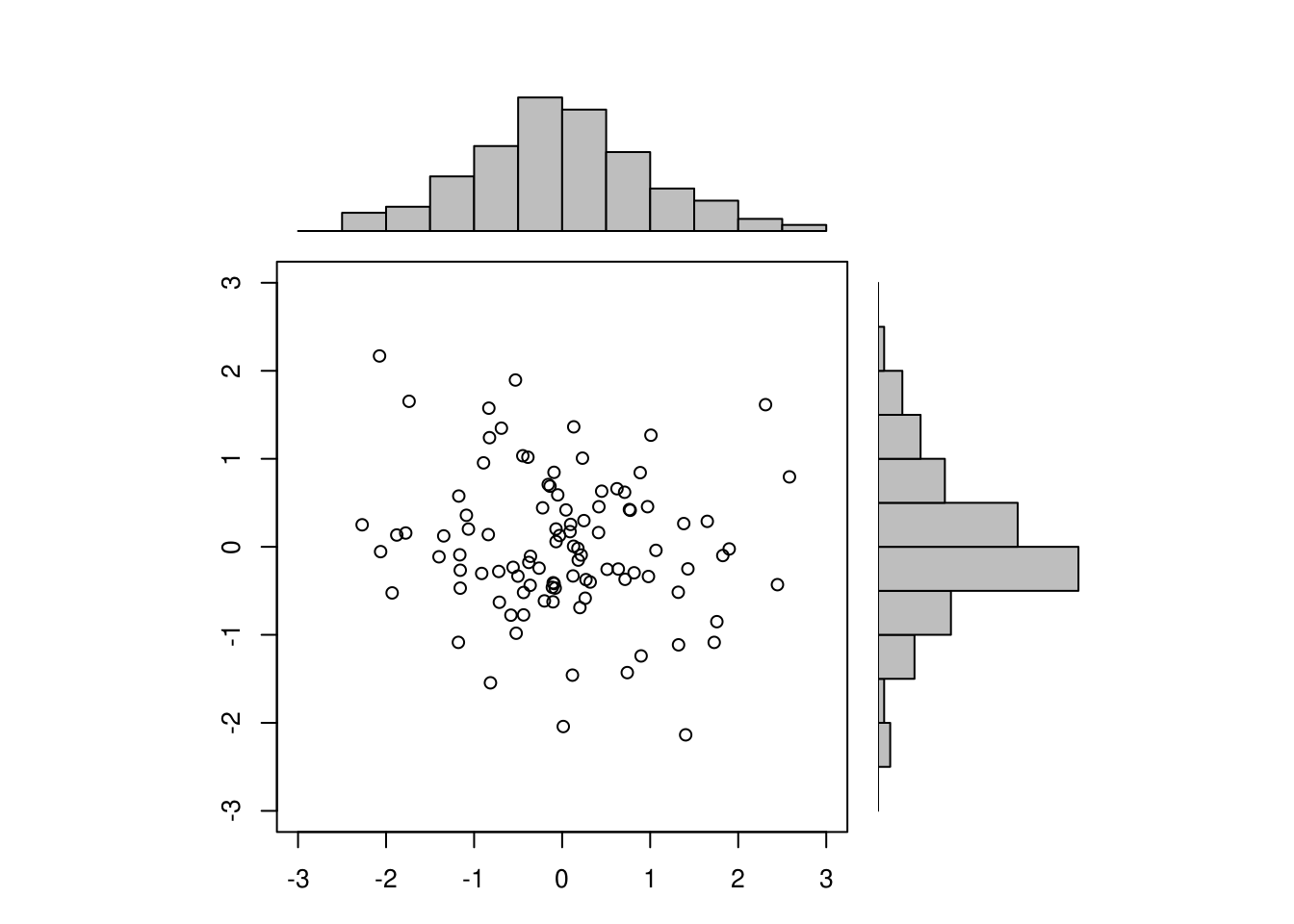par(fig = c(0, 0.8, 0, 0.8), new = TRUE)
plot(mtcars$wt, mtcars$mpg,
xlab = "Car Weight",
ylab = "Miles Per Gallon")
par(fig = c(0, 0.8, 0.55, 1), new = TRUE)
boxplot(mtcars$wt, horizontal = TRUE, axes = FALSE) par(fig = c(0.65, 1, 0, 0.8), new = TRUE) boxplot(mtcars$mpg, axes = FALSE)
mtext("Enhanced Scatterplot",
side = 3, outer = TRUE, line = -2)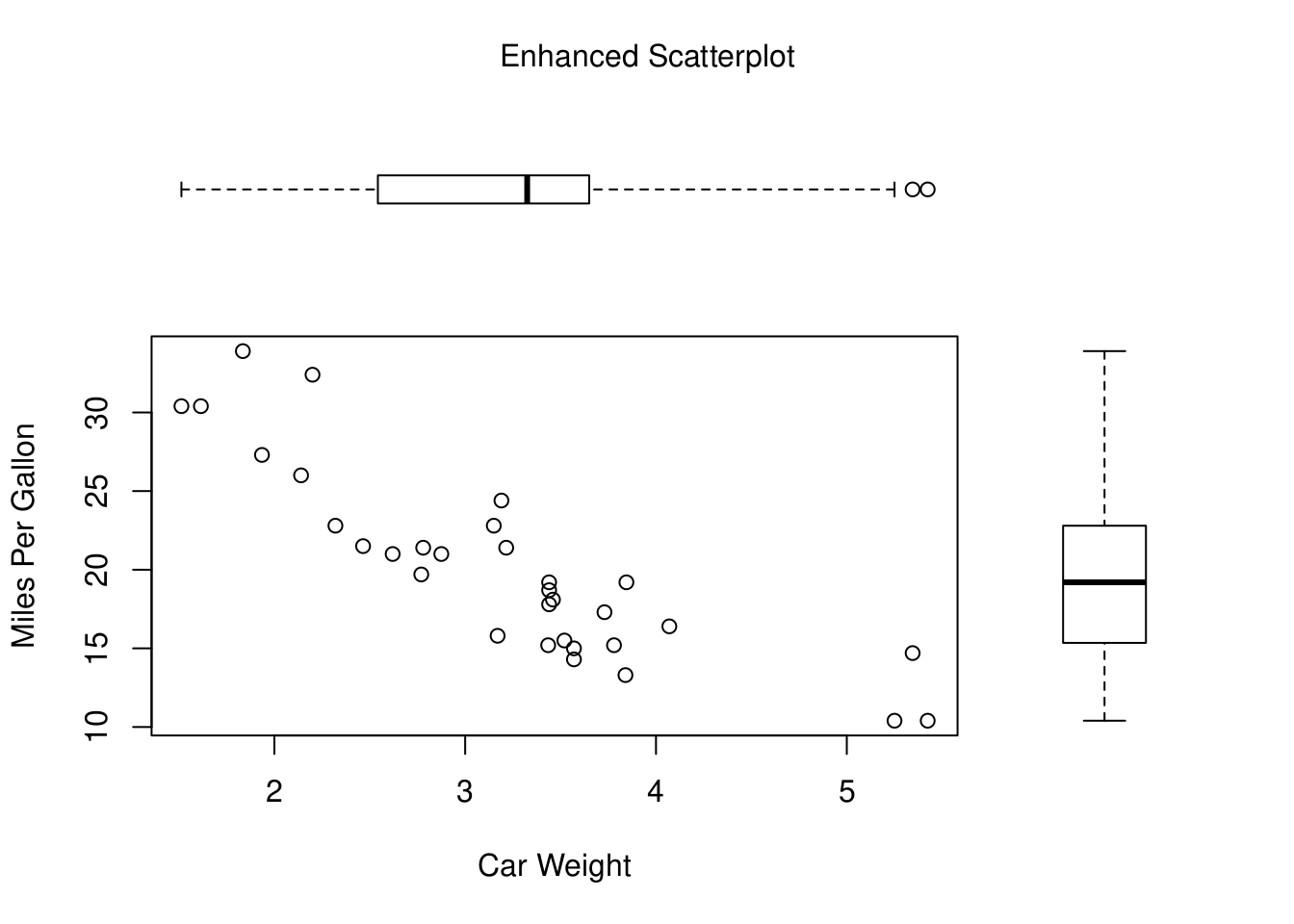fig 参数接受一个形如 c(x1, x2, y1, y2) 的 NDC (normalized device coordinates) 参数，比如上面的例子里 fig = c(0, 0.8, 0, 0.8) 表示这幅图的位置是 X 轴 c(0, 0.8) 和 Y 轴 c(0, 0.8)，即分别规定了宽度和高度及其对应的位置。